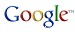VB Migration Partner

[HOWTO] Fix ReDim statements that change the rank of an array

VB6 permits to dynamically change the rank (i.e. the number of dimensions) of an array, as in this code snippet:

```        ' this array can have 1 or 2 dimensions
Dim arr() As Integer

Sub InitArray(ByVal rank As Integer)
Dim i As Integer, j As Integer

If rank = 1 Then
ReDim arr(100) As Integer
For i = 1 To UBound(arr)
arr(i) = i
Next
Else
ReDim arr(100, 20)
For i = 1 To UBound(arr, 1)
For j = 1 To UBound(arr, 2)
arr(i, j) =  i * j
Next
Next
End If
End Sub```

VB.NET don’t allow you to change the rank of an array, therefore the converted .NET code would raise one instance of the following error:

`  Number of indices exceeds the number of dimensions  in the indexed array`

for each statement where the array is accessed with two indexes. These is no definitive solution to this problem, however VB Migration Partner allows you to significantly decrease the number of this compilation error, using a combination of pragmas:

```        '## ParseReplace Dim arr() As Integer, arr2() As Integer
Dim arr() As Integer
'## arr2.ArrayRank 2
'## PreProcess "arr(?=\([^,)]+,[^,)]+\))", "arr2"
'## PreProcess "(?<=(LBound|UBound)\()arr(?=,)", "arr2"```

An explanation of each pragma is in order. The ParseReplace pragma causes VB Migration Partner to realize that there are actually two arrays (arr and arr2) and the ArrayRank pragma specifies that arr2 is a 2-dimensional array. The first subsequent PreProcess pragma changes arr into arr2 if the array reference is followed by two indexes that are separated by a comma – as in arr(1,2)  - whereas the second PreProcess pragma changes array references that appear in LBound and UBound method calls. The result is this piece of error-free .NET code:

```        ' VB.NET
Private arr() As Short
Private arr2(,) As Short

Public Sub InitArray(ByVal rank As Short)
Dim i As Short
Dim j As Short

If rank = 1 Then
ReDim arr(100)
For i = 1 To UBound6(arr)
arr(i) = i
Next
Else
ReDim arr2(100, 20)
For i = 1 To UBound6(arr2, 1)
For j = 1 To UBound6(arr2, 2)
arr2(i, j) =  i * j
Next
Next
End If
End Sub```

```        // C#
private short[] arr = null;
private short[,] arr2 = null;

public void InitArray(short rank)
short i = 0;
short j = 0;

if (rank == 1 )
{
arr = new short;
for (i = 1; i < = VB6Helpers.UBound(arr); i++)
{
arr[i] = i;
}
}
else
{
arr2 = new short[101, 21];
for (i = 1; i <= VB6Helpers.UBound(arr2, 1); i++)
{
for (j = 1; j <= VB6Helpers.UBound(arr2, 2); j++)
{
arr2[i, j] = i * j;
}
}
}
}
```
This approach isn't bulletproof, though. In fact, it works only for array occurrences whose indexes aren't function calls. For example, it doesn't work in the following case:

`        arr(GetIndex(1), GetIndex(2)) `

However,  you can easily take care of these residual errors by means of additional ParseReplace or PreProcess pragmas.

Follow Francesco Balena on VB6 migration’s group onsearch

Read Microsoft Corp’s official case study of a VB6 conversion using VB Migration Partner.

Code Architects and its partners offers remote and onsite migration services.

Subscribe to our free newsletter for useful VB6 migration tips and techniques.

A fully-working, time-limited Trial Edition of VB Migration Partner allows you to test it against your actual code

The price of VB Migration Partner depends on the size of the VB6 application, the type of license, and other factors

Migrating a VB6 application in 10 easy steps

Comparing VB Migration Partner with Upgrade Wizard

Migration tools: Feature Comparison Table